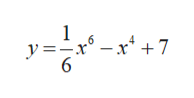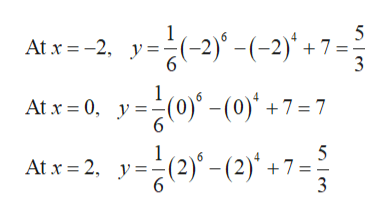Find the relative maxima, relative minima, horizontal points of inflection, and sketch the graph for y=1/6x^6-x^4+7

Question

Find the relative maxima, relative minima, horizontal points of inflection, and sketch the graph for

y=1/6x^6-x^4+7

Step 1

The given function is,help_outlineImage Transcriptionclose1 6 -x° y=_r°- r*+7 4 6 fullscreen
Step 2

Relative minimum and relative maximum:

Obtain the critical points as follows.

Differentiating the function with respect to x,help_outlineImage Transcriptionclosed1 dx6 6 x* + 7 .6x - 4x3 =r-4x Equate the above result to zero. x-4x3 0 (x2-4)0 (x+2(x-2)0 x = 0 or x2 = 0 or x- 2 = 0 x 0 or x 2 or x = 2 Thus, the critical points are -2, 0, and 2 fullscreen
Step 3

Find the values of y of the corresponding values...help_outlineImage Transcriptionclose1 5 6 At x 2 y(-2)° -(-2) +7 =; 3 1 At x 0, y(0) -(0)+7 = 7 6 1 5 6 At x 2, y(2) - (2)' +7 = 6 fullscreen

Want to see the full answer?

See Solution

Want to see this answer and more?

Our solutions are written by experts, many with advanced degrees, and available 24/7

See Solution
Tagged in

Other